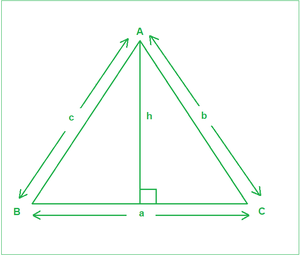# Sine Rule with Derivation, Example and Implementation

• Last Updated : 13 Jul, 2021

Given angles(in degrees) A, C, and the side c, corresponding to the figure below, the task is to find the remaining two sides a and b.Attention reader! All those who say programming isn't for kids, just haven't met the right mentors yet. Join the  Demo Class for First Step to Coding Coursespecifically designed for students of class 8 to 12.

The students will get to learn more about the world of programming in these free classes which will definitely help them in making a wise career choice in the future.

Examples:

Input: A = 45, C = 35, c = 23
Output:
28.35
39.49
Explanation:
a is 28.35 and b is 39.49

Input: A = 45, C = 45, c = 10
Output:
10
14.14

Approach: The idea is to use Sine rule. It states that the sides of any triangle are proportional to the sine of the angles opposite to them. a / Sin(A) = b / Sin(B) = c / Sin(C). The derivation is described below:

As is evident from the figure above:

A perpendicular of length h has been drawn on BC from A. From General trigonometric rules:

SinB=h/c——–(1)

SinC=h/b——–(2)

From the above two equations, we get:

c x SinB=b x SinC

Or b/SinB=c/SinC—–(3)

Similarly, if a perpendicular is drawn from B to AC, we can get:

a/SinA=c/SinC——-(4)

From Equations (3) and (4), we get:

a/SinA=b/SinB=c/SinC

Follow the steps below to solve the problem:

• Change the angles A and C from degrees to radians to be able to be used in the inbuilt functions.
• Calculate the angle B using the observation that sums of angles of a triangle sums up to 180 degrees.
• Use the Sine rule to calculate the sides a and b.

Below is the implementation of the above approach:

## C++14

 `// C++ program for the above approach``#include ``using` `namespace` `std;` `// Function to calculate remaining two sides``void` `findSides(``double` `A, ``double` `C, ``double` `c)``{``    ``// Calculate angle B``    ``double` `B = 180 - (A + C);` `    ``// Convert angles to their respective radians for``    ``// using trigonometric functions``    ``A = A * (3.14159 / 180);``    ``C = C * (3.14159 / 180);``    ``B = B * (3.14159 / 180);` `    ``// Sine rule``    ``double` `a = (c / ``sin``(C)) * ``sin``(A);``    ``double` `b = (c / ``sin``(C)) * ``sin``(B);` `    ``// Precision of 2 decimal spaces``    ``cout << fixed << setprecision(2);` `    ``// Print the answer``    ``cout << a << endl;``    ``cout << b << endl;``}` `// Driver Code``int` `main()``{``    ``// Input``    ``double` `A = 45.0;``    ``double` `C = 35.0;``    ``double` `c = 23;` `    ``// Function Call``    ``findSides(A, C, c);``    ``return` `0;``}`

## Java

 `// Java program for the above approach``class` `GFG{` `// Function to calculate remaining two sides``static` `void` `findSides(``double` `A, ``double` `C,``                      ``double` `c)``{``    ` `    ``// Calculate angle B``    ``double` `B = ``180` `- (A + C);` `    ``// Convert angles to their respective``    ``// radians for using trigonometric functions``    ``A = A * (``3.14159` `/ ``180``);``    ``C = C * (``3.14159` `/ ``180``);``    ``B = B * (``3.14159` `/ ``180``);` `    ``// Sine rule``    ``double` `a = (c / Math.sin(C)) * Math.sin(A);``    ``double` `b = (c / Math.sin(C)) * Math.sin(B);` `    ``// Print the answer``    ``System.out.println(String.format(``"%.2f"``, a));``    ``System.out.println(String.format(``"%.2f"``, b));``}` `// Driver code``public` `static` `void` `main(String[] args)``{``    ` `    ``// Input``    ``double` `A = ``45.0``;``    ``double` `C = ``35.0``;``    ``double` `c = ``23``;` `    ``// Function Call``    ``findSides(A, C, c);``}``}` `// This code is contributed by abhinavjain194`

## Python3

 `# Python3 program for the above approach``import` `math` `# Function to calculate remaining two sides``def` `findSides(A, C, c):``    ` `    ``# Calculate angle B``    ``B ``=` `180` `-` `(A ``+` `C)` `    ``# Convert angles to their respective radians``    ``# for using trigonometric functions``    ``A ``=` `A ``*` `(``3.14159` `/` `180``)``    ``C ``=` `C ``*` `(``3.14159` `/` `180``)``    ``B ``=` `B ``*` `(``3.14159` `/` `180``)` `    ``# Sine rule``    ``a ``=` `(c ``/` `math.sin(C)) ``*` `math.sin(A)``    ``b ``=` `(c ``/` `math.sin(C)) ``*` `math.sin(B)` `    ``# Precision of 2 decimal spaces` `    ``# Print the answer``    ``print``(``"{0:.2f}"``.``format``(a))``    ``print``(``"{0:.2f}"``.``format``(b))` `# Driver Code` `# Input``A ``=` `45.0``C ``=` `35.0``c ``=` `23` `# Function Call``findSides(A, C, c)` `# This code is contributed by target_2`

## C#

 `// C# program for the above approach``using` `System;``class` `GFG{` `// Function to calculate remaining two sides``static` `void` `findSides(``double` `A, ``double` `C,``                      ``double` `c)``{``    ` `    ``// Calculate angle B``    ``double` `B = 180 - (A + C);` `    ``// Convert angles to their respective``    ``// radians for using trigonometric functions``    ``A = A * (3.14159 / 180);``    ``C = C * (3.14159 / 180);``    ``B = B * (3.14159 / 180);` `    ``// Sine rule``    ``double` `a = (c / Math.Sin(C)) * Math.Sin(A);``    ``double` `b = (c / Math.Sin(C)) * Math.Sin(B);` `    ``// Print the answer``    ``Console.WriteLine(``"{0:F2}"``,a);``    ``Console.WriteLine(``"{0:F2}"``,b);``}` `// Driver code``public` `static` `void` `Main(String[] args)``{``    ` `    ``// Input``    ``double` `A = 45.0;``    ``double` `C = 35.0;``    ``double` `c = 23;` `    ``// Function Call``    ``findSides(A, C, c);``}``}` `// This code is contributed by shivanisinghss2110`

## Javascript

 ``
Output
```28.35
39.49```

Time Complexity: O(1)
Auxiliary Space: O(1)

My Personal Notes arrow_drop_up